• Hash256
• Hash160
• Reverse Bytes
• Satoshis

# Difficulty

A mechanism for regulating the time it takes to mine a block.

## What is the difficulty?

The difficulty is a number that regulates how long it takes for miners to add new blocks of transactions to the blockchain.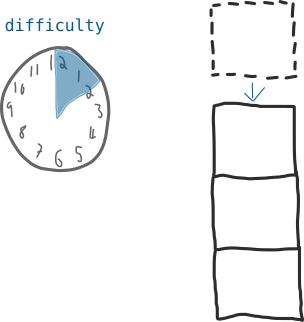This difficulty value updates every 2 weeks to ensure that it takes 10 minutes (on average) to add a new block to the blockchain.

## Why is the difficulty important?

Because it ensures that blocks of transactions are added to the blockchain at regular intervals, even as more miners join the network.

If the difficulty remained the same, it would take less time between adding new blocks to the blockchain as new miners join the network.

## When does the difficulty change?

The difficulty adjusts every 2016 blocks (roughly every 2 weeks).

At this interval, each node takes the expected time for these 2016 blocks to be mined (2016 x 10 minutes), and divides it by the actual time it took (however many minutes):

``````expected / actual
20160 / actual``````

If miners were able to solve each block more quickly than expected; say 9 minutes per block for example, you’d get a number like this

``20160 / 18144 = 1.11``

Each node then uses this number to adjust the difficulty for the next 2016 blocks:

``difficulty x 1.11 = new difficulty``
• If the number is greater than 1 (i.e. blocks were mined quicker than expected), the difficulty increases.
• If the number is less than 1 (i.e. blocks were mined slower than expected) the difficulty decreases.

And that’s it. Every miner on the bitcoin network now works with this new difficulty for the next 2016 blocks.

The difficulty will only adjust by a factor of 4 at most (i.e. a number not greater than 4 or less than 0.25). This is to prevent abrupt changes from one difficulty to the next.

## How does the difficulty control time between blocks?

Okay, I’ll start with a simple example and go from there.

### 1. Simple example

Let’s say I give you a range of numbers from 1 to 100.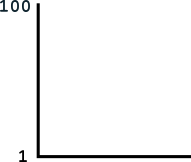Now, you are able to randomly generate a number between 1 and 100 once every minute. And your goal is to generate a number below my target number.

So let’s say I set the target at 50: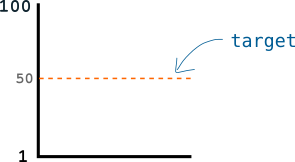Seeing as you’re only able to generate a number between 1 and 100 once a minute, this should take you 2 minutes.

But that’s too easy. Now I lower the target to 20, which means you’re only going to be able to generate a winning number 1/5 of the time, or once every 5 minutes: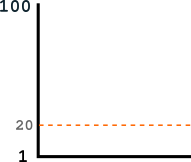The lower the target, the more difficult it gets to generate a winning number.

It’s not going to be 5 minutes every time because you could get lucky with the first number you generate. But over the long run it will work out to be 5-minute intervals.

Therefore, based on how many numbers you are able to generate per minute, I can use the height of the target to control how long it takes you to find a winning number.

#### Introducing the difficulty…

I’m a computer, and instead of telling you the target value directly, I find it easier to give you the target by dividing the range of numbers with a new number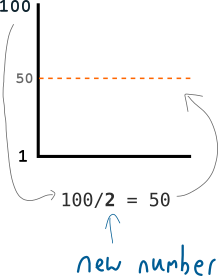This new number is able to control the height of the target.

This new number is the difficulty, and it’s used as an easy way for me to modify the height of the target.

Here’s the equation for finding the target:

``target = targetmax / difficulty``

Furthermore, I can use this difficulty value to help me set the target to any level I want: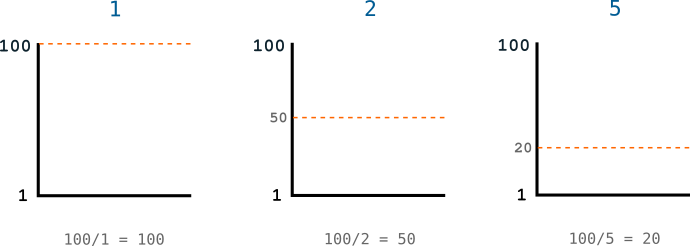The higher the difficulty, the lower the target.

So I use the difficulty to control the target, and therefore how long it takes for you to generate a winning number.

### 2. Bitcoin example.

The difficulty in bitcoin works in exactly the same way – it’s used to set a target value, and miners keep generating numbers (hashing their candidate blocks) in the hope that they will find a number lower than this target value: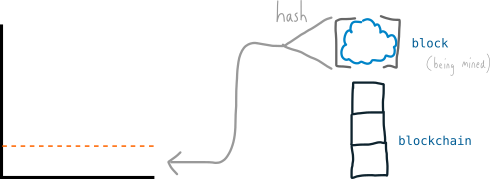And seeing as miners are able to generate thousands of numbers (hash values) per minute, bitcoin uses ridiculously big numbers: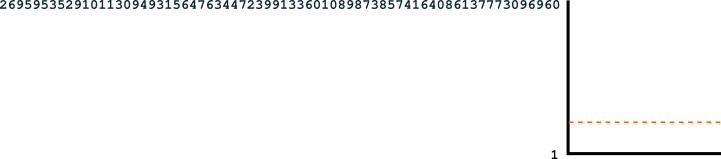The numbers in bitcoin are just on a much bigger scale.

And due to the fact that there are now thousands of miners trying to find winning numbers, to ensure that a winning number is found every 10 minutes (instead of every few seconds), the range of successful numbers ends up being absolutely tiny: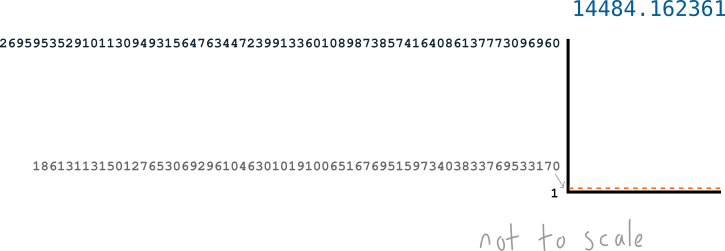Even though that difficulty number looks big, the target is still absurdly difficult to get under. It’s like a lottery.

#### Introducing hexadecimal numbers…

Because these target numbers are so big, computers prefer to work with them in hexadecimal format.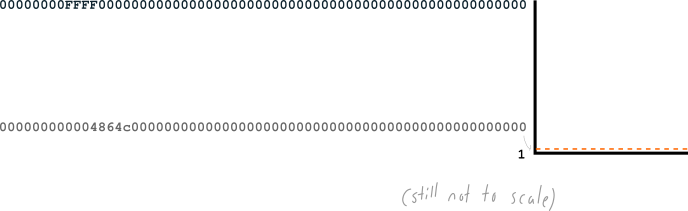That’s why the hash values for blocks look like this: `000000000003ba27aa200b1cecaad478d2b00432346c3f1f3986da1afd33e506` – it’s hexadecimal.

And even though it’s got letters in there, it’s still a number. So the target is a hexadecimal value, and miners are trying to get a hexadecimal hash value below the target.

In fact, you can easily convert between hexadecimal and “normal numbers” (better known as decimal numbers):

So that’s why you usually see the hash and the target as bunches of numbers and letters – they’re in hexadecimal as opposed to decimal (which is what humans are more familiar with). But just remember that both these decimal and hexadecimal numbers have the same value, and you can easily convert between the two.

Awkwardly, the difficulty is usually given in decimal format, whereas the hashes and targets are stored in hexadecimal. But as I say, they’re both numbers, and as long as you convert them to the same format you can still work with them.

## Example: Finding the target using the difficulty.

Let’s work out target for block 100,000 using the difficulty. But let’s do it mostly in decimal numbers, because they’re easier to understand.

Here’s the difficulty for block 100,000 (as found in the block header):

``````{
"height" : 100000,
"difficulty" : 14484.16236123,
}``````

Lovely.

Now, let’s note down the equation we’re going to use to find the target:

``target = targetmax / difficulty``

And let’s get the targetmax and difficulty ready to insert it in to the equation.

``````targetmax = 0x00000000FFFF0000000000000000000000000000000000000000000000000000
difficulty = 14484.162361``````
• The targetmax is a fixed value, and it can be found here.
• I got the difficulty from the block header information I pasted above.

The targetmax is currently in hexadecimal format though, so let’s convert that to decimal.

``targetmax = 26959535291011309493156476344723991336010898738574164086137773096960``

I used this hexadecimal to decimal converter to do this.

I know targetmax is a hexadecimal number because it starts with 0x, which is a prefix to signify that what comes next is hexadecimal (the 0x isn’t part of the number, it’s just a note). Plus the presence of letters within the value is a dead giveaway anyway.

Now we can just plug these numbers in to the equation and away we go:

``````target = targetmax / difficulty
target = 26959535291011309493156476344723991336010898738574164086137773096960 / 14484.162361
target = 1861311315012765306929610463010191006516769515973403833769533170``````

Ta da.

So when the miner was trying to solve block 100,000, she wanted to get a hash for her candidate block that would be below `1861311315012765306929610463010191006516769515973403833769533170`.

### Check it…

Let’s compare this with the hash she got for the block to check that she was genuinely successful (i.e. her hash for the block was below the target):

``````target = 1861311315012765306929610463010191006516769515973403833769533170

Oh yeah, the hash is in hexadecimal format. Sorry. Let me convert from hexadecimal to decimal again so that we can compare the two numbers:

``````target = 1861311315012765306929610463010191006516769515973403833769533170
hash   = 1533267872647776902154320487930659211795065581998445848740226310``````

Yep, that hash is a smidgin smaller than the target. But it is lower, so the hash is successful and the block can be added to the blockchain.

Both the target and block hash would be stored as hexadecimal numbers inside the block header, so here’s what they look like if we convert them both back to hexadecimal:

``````target = 4864C00004D6AC7CD33F734B8EB28B24729FE151953EC57A21EF2

## Where can I find the current difficulty?

You can find the current difficulty by entering the `getdifficulty` command in to your bitcoin client:

The difficulty can also be found with `getmininginfo` (amongst other mining info).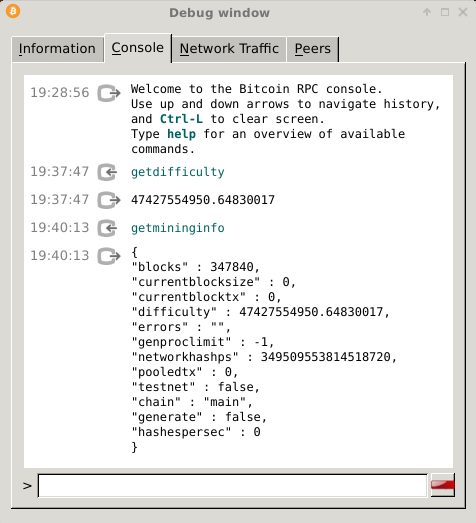Hey there, it's Greg.

I'll let you know about cool website updates, or if something seriously interesting happens in bitcoin.

Don't worry, it doesn't happen very often.